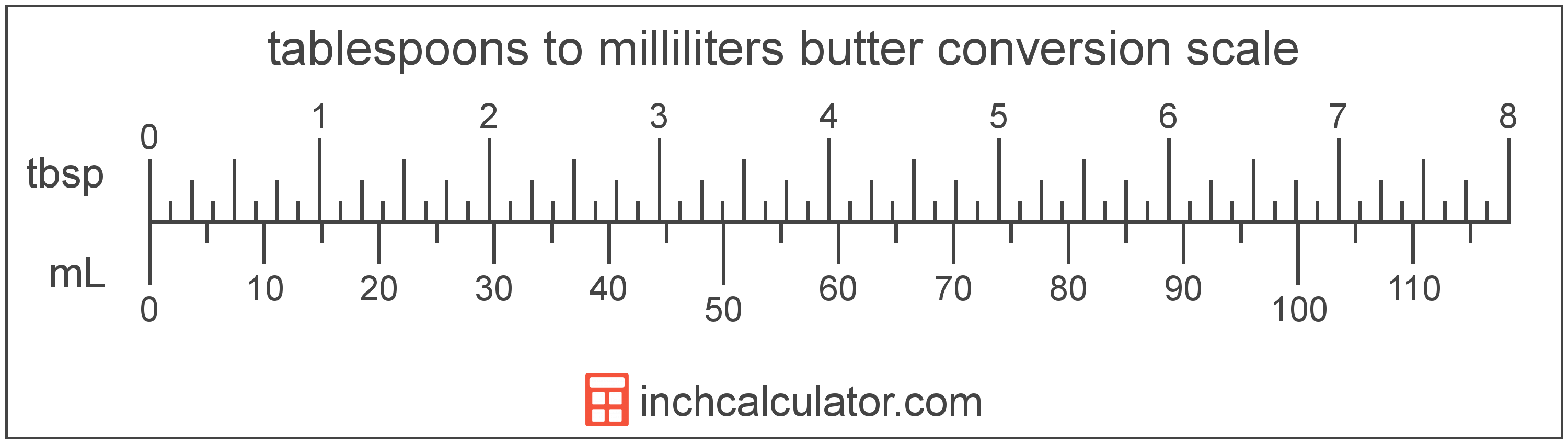# Convert Milliliters of Butter to Tablespoons

Enter the butter in milliliters below to get the value converted to tablespoons.

Results in Tablespoons:1 mL = 0.067628 tbsp

Do you want to convert tablespoons to milliliters?

## How to Convert Milliliters to Tablespoons

To convert a measurement in milliliters to a measurement in tablespoons, multiply the butter by the following conversion ratio: 0.067628 tablespoons/milliliter.

Since one milliliter of butter is equal to 0.067628 tablespoons, you can use this simple formula to convert:

tablespoons = milliliters × 0.067628

The butter in tablespoons is equal to the butter in milliliters multiplied by 0.067628.

For example, here's how to convert 5 milliliters to tablespoons using the formula above.
tablespoons = (5 mL × 0.067628) = 0.33814 tbsp## What Is a Milliliter?

Butter is sometimes measured by volume in milliliters. One stick of butter contains about 118.3 milliliters.

The milliliter is an SI unit of volume in the metric system. A milliliter is sometimes also referred to as a millilitre. Milliliters can be abbreviated as mL; for example, 1 milliliter can be written as 1 mL.

## What Is a Tablespoon?

One tablespoon of butter is equal to 1/8 of a stick or 1/2 ounce.

The tablespoon is a US customary unit of volume. Tablespoons can be abbreviated as tbsp, and are also sometimes abbreviated as T, Tbls, or Tb. For example, 1 tablespoon can be written as 1 tbsp, 1 T, 1 Tbls, or 1 Tb.

## Milliliter to Tablespoon Conversion Table

Table showing various milliliter measurements converted to tablespoons.
Milliliters Tablespoons
1 mL 0.067628 tbsp
2 mL 0.135256 tbsp
3 mL 0.202884 tbsp
4 mL 0.270512 tbsp
5 mL 0.33814 tbsp
6 mL 0.405768 tbsp
7 mL 0.473396 tbsp
8 mL 0.541024 tbsp
9 mL 0.608652 tbsp
10 mL 0.67628 tbsp
11 mL 0.743908 tbsp
12 mL 0.811537 tbsp
13 mL 0.879165 tbsp
14 mL 0.946793 tbsp
15 mL 1.0144 tbsp
16 mL 1.082 tbsp
17 mL 1.1497 tbsp
18 mL 1.2173 tbsp
19 mL 1.2849 tbsp
20 mL 1.3526 tbsp
21 mL 1.4202 tbsp
22 mL 1.4878 tbsp
23 mL 1.5554 tbsp
24 mL 1.6231 tbsp
25 mL 1.6907 tbsp
26 mL 1.7583 tbsp
27 mL 1.826 tbsp
28 mL 1.8936 tbsp
29 mL 1.9612 tbsp
30 mL 2.0288 tbsp
31 mL 2.0965 tbsp
32 mL 2.1641 tbsp
33 mL 2.2317 tbsp
34 mL 2.2994 tbsp
35 mL 2.367 tbsp
36 mL 2.4346 tbsp
37 mL 2.5022 tbsp
38 mL 2.5699 tbsp
39 mL 2.6375 tbsp
40 mL 2.7051 tbsp